# Writing a simple Volatility plugin - Part 1

This post covers the basics of writing a simple plugin for the Volatility framework using python.

## Prerequisites

• Knowledge of programming in python (Using functions, classes etc…)
• Experience with using volatility

It is not really necessary to have a very good experience with volatility to understand this blog post but I recommend it as it will be easier to “know what you’re doing”.

## Volatility? what is it?

This section is primarily for people who have never used volatility before, so I will give a brief introduction of the tool. Volatility is an open source memory forensics framework helpful in analyzing memory/RAM dumps.

Volatility is a very robust and extremely popular tool when it comes to forensic investigation of memory images.

## Writing the plugin

In this post, we will go through a step by step procedure of writing a custom pslist (used to enumerate active/running processes) plugin.

To start we will create a skeleton of the plugin with a simple “Hello world!” before proceeding to the more complex parts.

### Creating plugin folder

$mkdir testplugin$ cd testplugin
$touch testplugin.py  Open the python file just created using any text editor you want. I prefer VS Code. ### Getting the foundation right Before proceeding to the complex coding part, we’ll approach this by writing a simple volatility plugin which just prints “Hello world!”. So below is the code for the program, import volatility.plugins.common as common class TestPlugin(common.AbstractWindowsCommand): """Prints Hello world!""" def render_text(self, outfd, data): outfd.write("Hello world!\n")  Note: All the python code written in this blog is of python 2 #### Understanding the code Having written the above code, let us try to understand what it is line by line. • import volatility.plugins.common • Used to import the common library which is a part of volatility’s framework • class TestPlugin(common.AbstractWindowsCommand) • Every plugin that you use in volatility is actually a python class. Python classes contain the code that determines the working of the plugin. • As you can observe, we are inheriting one of the command classes. In this case, AbstractWindowsCommand. • Inheriting this class is what actually gives this class the definition of a plugin. Otherwise, it is just another python class. • def render_text(self, outfd, data) • The codebase/framework of volatility is designed in such a way that the Volatility always looks for the render_X() function where X indicates the output parameter or the format of result produced by the plugin. • outfd is the output file descriptor. It specifies the output stream to which the result is written. In this case, it is stdout. • data is actually the output of another method called calculate. The calculate method actually computes the result and passes it to render_text() which writes it to stdout. #### Testing output Now that we understood how the above code works, let us run it and check if it works. Before testing the plugin, let us see if volatility recognizes our plugin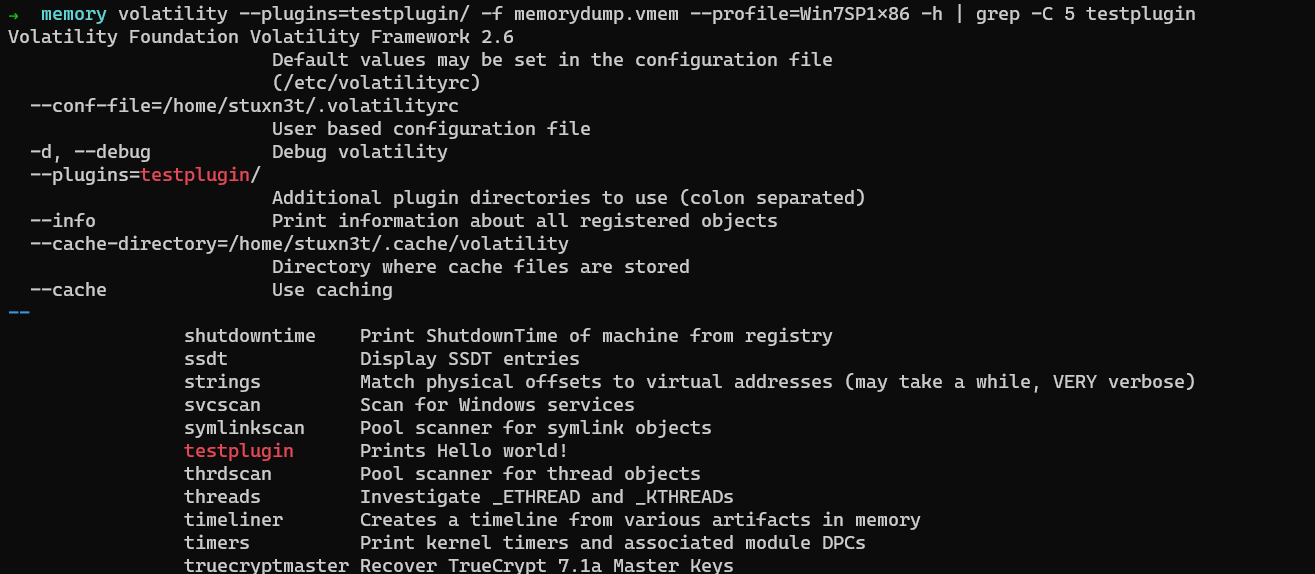Yes! it is successfully recognized. Now let us run it.### Building the pslist plugin Now that we know how to write the basic skeleton of a plugin, we need it do something with the memory dump i.e extract some piece of evidence. So here we will try to write a plugin which enumerates all the active processes running in the system. Normally, you can see the running processes using the Task Manager.  import volatility.plugins.common as common import volatility.utils as utils import volatility.win32 as win32 class TestPlugin(common.AbstractWindowsCommand): """ Works exactly like pslist """ def calculate(self): addr_space = utils.load_as(self._config) tasks = win32.tasks.pslist(addr_space) return tasks def render_text(self, outfd, data): for tasks in data: PID = tasks.UniqueProcessId CreateTime = tasks.CreateTime Process_name = tasks.ImageFileName outfd.write("{0}\t {1}\t {2}\n".format(PID, CreateTime, Process_name))  ### Understanding the code • We have imported 2 new libraries. • volatility.utils • volatility.win32 • def calculate(self) • As discussed, calculate method is required to compute the data needed and pass the result to render_text() function • addr_space = utils.load_as(self._config) • We are here declaring a variable which will contain the address space identified by self_.config • address space is actually generated from the memory dump we supply to volatility. • win32.tasks.pslist(addr_space) • Using the address space, we enumerate the processes. • This is done by looking for process objects This completes the explanation for the calculate() function. Now we move to discuss the terms • UniqueProcessId • PID of a process. Every process has a unique ID with which it can recognized distinctly. • CreateTime • The timestamp of when the process was executed. • ImageFileName • The name of the process. Ex: svchost.exe, chrome.exe, notepad.exe These terms are associated and are fields inside the _EPROCESS structure in Windows. _EPROCESS is a data structure in windows which contains various details associated with a process. What we are essentially doing using the above python code is that we are iterating over the doubly-linked list through which each _EPROCESS is connected and retrieving the necessary evidence we need. Below is the screenshot showing various other fields in the _EPROCESS structure using livekd CreateTime Field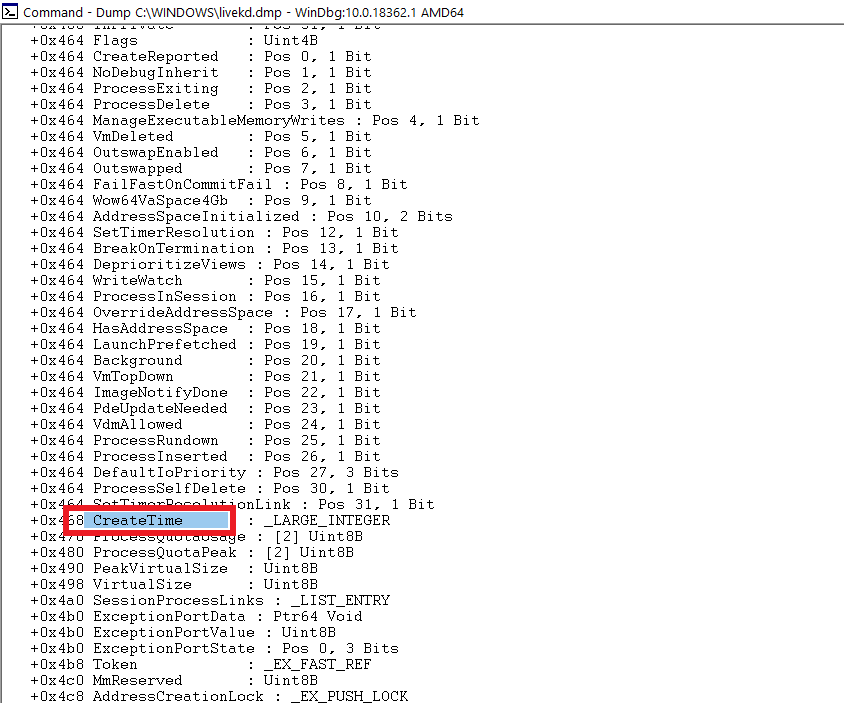UniqueProcessId Field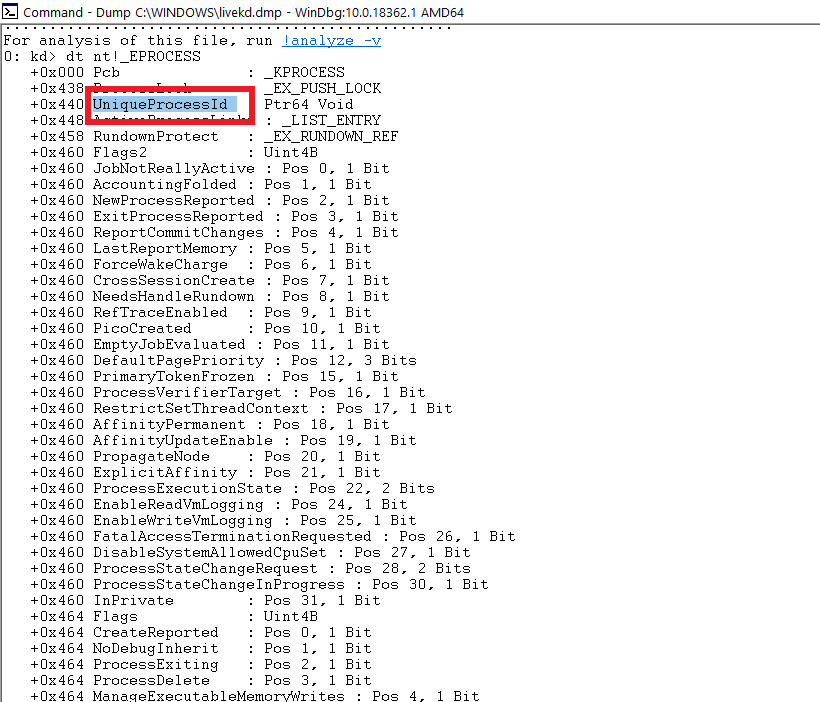ImageFileName Field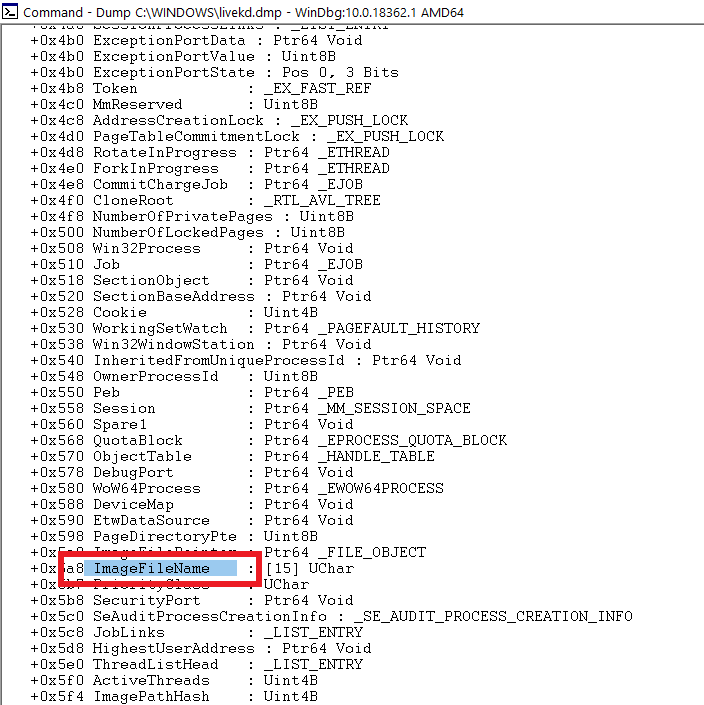Other interesting and useful fields are • ExitTime • The timestamp of the event when the process finishes execution. • InheritedFromUniqueProcessId • PID of the parent process. And many more… Now that we’ve written our plugin and how it works, let us test it by running it. #### Testing output $ volatility --plugins=testplugin/ -f memorydump.vmem --profile=Win7SP1x86 testplugin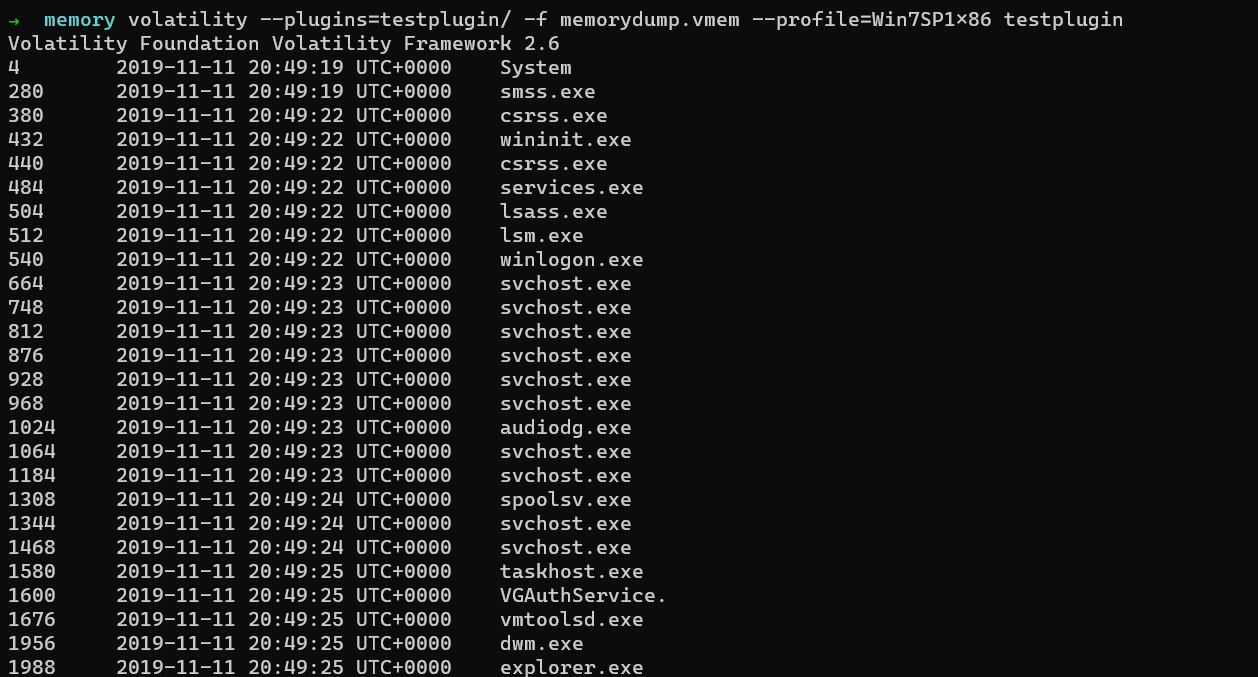So our plugin works perfectly as expected.

## Final thoughts

This blogpost was a very simple introduction to writing plugins in volatility. The real ones that volatility uses are a lot complex since they have lot of things to handle like

• Error handling
• Plugin must be efficient

So, plugin developers at Volatility need to keep these things in mind before they release a plugin. However, now that you have understood the basics required, it will be a lot easier to read the original plugin code from volatility’s code base.

• What is EPROCESS?
• Volatility’s pstree